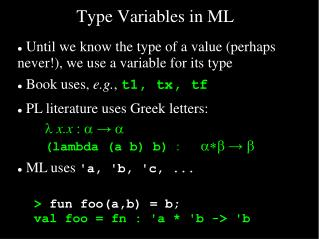DownloadDownload PresentationType Variables in ML

# Type Variables in ML

Download Presentation## Type Variables in ML

- - - - - - - - - - - - - - - - - - - - - - - - - - - E N D - - - - - - - - - - - - - - - - - - - - - - - - - - -
##### Presentation Transcript

1. Type Variables in ML • Until we know the type of a value (perhaps never!), we use a variable for its type • Book uses, e.g., t1, tx, tf • PL literature uses Greek letters: • l x.x : a→a • (lambda (a b) b) : a*b→b • ML uses 'a, 'b, 'c, ... > fun foo(a,b) = b; val foo = fn : 'a * 'b -> 'b

2. sum: int list -> int • member: 'a * 'a list -> bool ML: Let's Write... Recall: fun reverse(nil) = nil | reverse(x::t) = reverse(t) @ [x];

3. ML: Local environments using let • Recall (?) mergesort: Divide-and-conquer for sorting a list • Split list into two sorted sublists, then merge them: merge([1,3,5], [2,4,6]) = [1,2,3,4,5,6] • So first write merge:

4. ML: Local environments using let > fun merge(nil,M) = M | merge(L,nil) = L | merge(x::xs, y::ys) = if (x:int) < y then x::merge(xs,y::ys) else y::merge(x::xs,ys); val merge = fn : int list * int list -> int list > merge([1,3,5], [2,4,6]); val it = [1,2,3,4,5,6] : int list Now write split:

5. > fun split(nil) = (nil,nil) | split([a]) = ([a], nil) | split(a::b::cs) = let val (M,N) = split(cs) in (a::M, b::N) end; val split = fn : 'a list -> 'a list * 'a list > split([1,2,3,4,5]); val it = ([1,3,5],[2,4]) : int list * int list Finally write mergeSort:

6. > fun mergeSort(nil) = nil | mergeSort([a]) = [a] | mergeSort(L) = let val (M,N) = split(L); val M = mergeSort(M); val N = mergeSort(N) in merge(M,N) end; val mergeSort = fn : int list -> int list > mergeSort([5,4,3,2,1]); val it = [1,2,3,4,5] : int list

7. ML: Polymorphism • Note that merge is the only function here explicitly declaring a type (int): fun merge(nil,M) = M | merge(L,nil) = L | merge(x::xs, y::ys) = if (x:int) < y then x::merge(xs,y::ys) else y::merge(x::xs,ys); • We can “factor out” the int comparison, and make merge and mergeSort polymorphic:

8. ML: Polymorphism > fun merge(nil,M,C) = M (* C is comparator fun *) | merge(L,nil,C) = L | merge(x::xs, y::ys,C) = if C(x,y) then x::merge(xs,y::ys,C) else y::merge(x::xs,ys,C); val merge = fn : 'a list * 'a list * ('a * 'a -> bool) -> 'a list

9. ML: Polymorphism > fun merge Sort(nil,C) = nil | mergeSort([a],C) = [a] | mergeSort(L,C) = let val (M,N) = split(L); val M = mergeSort(M,C); val N = mergeSort(N,C) in merge(M,N,C) end; val mergeSort = fn : 'a list * ('a * 'a -> bool) -> 'a list

10. ML: Polymorphism > fun intcmp(a:int, b) = a < b; val intcmp = fn : int * int -> bool > fun realcmp(a:real, b) = a < b; val realcmp = fn : real * real -> bool > mergeSort([5,4,3,2,1], intcmp); val it = [1,2,3,4,5] : int list > mergeSort([5.5, 4.4, 3.3, 2.2, 1.1], realcmp); val it = [1.1,2.2,3.3,4.4,5.5] : real list

11. ML: Polymorphism • Q.: What's the point? • A.: ML supports functions as first -class objects (as in Scheme), while also providing strong type-checking (as in Java) • Java supports this ability (abstract comparison) through the Comparable interface.